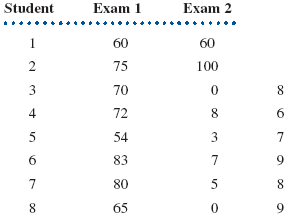Chat Now

# In a large introductory sociology course a professor gives two exams The professor wants to determine whether the scores students receive on the second exam are correlated with their scores on the

### In a large introductory sociology course a professor gives two exams The professor wants to determine whether the scores students receive on the second exam are correlated with their scores on the

In a large introductory sociology course, a professor gives two exams. The professor wants to determine whether the scores students receive on the second exam are correlated with their scores on the first exam. To make the calculations easier, a sample of eight students is selected. Their scores are shown in the accompanying table.a. Construct a scatter plot of the data, using exam 1 score as the variable. Does the relationship look linear?

b. Assuming a linear relationship exists between scores on the two exams, compute the value for Pearson r.

c. How well does the relationship account for the scores on exam 2?

mahesh 24-Aug-2020Get solution